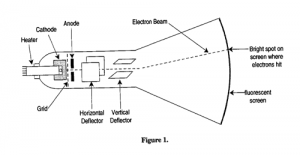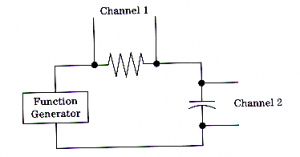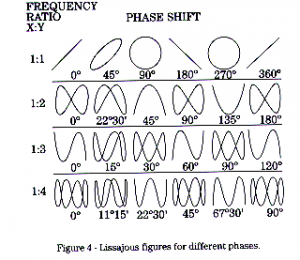# How to Test Instruments in your Lab

Learn here how to use some common test instruments in your research laboratory. The common type of instruments we are using in our lab are oscilloscope, digital multimeter, frequency counter, function generator, LCR meter, and power supplies.

## Oscilloscope testing

The oscilloscope is one of the most used test and measurement tools in laboratory.  It is really a type of analog voltmeter with an arbitrary zero. It can read DC voltages as an offset voltage as well as AC voltages by displaying the true waveform. Most modern oscilloscopes are capable of measuring AC signals over a wide range of frequencies and you should check your oscilloscope for its frequency response.  The key component of the oscilloscope is a cathode ray tube shown in Fig. 1.  Here a beam of electrons is ejected from the “electron gun” and is directed to the center of the screen.  The screen is covered with a luminescent material emitting light where the electron beam strikes it.  The light emission lasts a fraction of a second after the impact (an effect known as “afterglow”). The oscilloscope is equipped with a vertical pair of plates for deflecting the electron beam in the horizontal direction, and with a horizontal pair for deflecting in the vertical direction. When an input signal to be measured is applied to the horizontal plates, the vertical distance that the actual beam spot moves from the center of the screen becomes proportional to the applied voltage V.  The vertical plates are often connected to a known time varying voltage.  An example is the ‘’saw-tooth” signal, which linearly increases with time up to a set limit and then very quickly drops back to zero.  In this way, one can display the waveform of the input signal as a function of time.

##Function Generator testing

This device produces sine, square, and triangle waves plus a few others that you will not need for this experiment. The frequency range varies from less than 1 Hz to many MHz. You will use this generator to provide an AC signal to your circuits and the oscilloscope. This will enable you to study different waveforms and to get an understanding of some basic AC signals.   There are several function generators available in the laboratory.  Some of them have a synchronous pulse or square wave output useful for triggering purpose and a voltage-controlled oscillator VCO, which allows one to vary the frequency by applying a controlled-voltage to a connector on the instrument.

## Digital Multimeter (DMM)

Digital multimeter is a versatile instrument used for measuring voltage, current, and resistance as well as the circuit continuity. The instrument has very high input impedance so that it is virtually not seen by the circuit under test. That is, it does not impose any significant load on the circuit.   There are several digital multimeters available in the laboratory.  They differ in the sensitivity and accuracy for the voltage, current, and resistance measurements.

## Frequency Counter/Timer

Frequency counter/timer is used for measuring and analyzing frequency, phase, and time-interval signal characteristics.  If you are dealing with a source of unknown frequency, it is wise to check the frequency from the counter against a crude measurement of the period of the waveform on the oscilloscope.  The frequency counter DC 504 used in the laboratory is easy to use and it works reliably if it is triggering properly.  If a frequency counter is not triggering correctly, the reading will be very wrong (e.g., the frequency is off by a factor of exactly two) and unstable.

## LRC Meter

This instrument is essentially an impedance bridge, which can be used to measure the values of reactive components, such as a capacitor and an inductor.  It can also be used for the resistance measurements, but they are more conveniently done by a digital multimeter.  The LRC meter is used to measure the actual (as opposed to nominal) value of fixed capacitors or inductors before inserting them into a circuit.

Procedure:  Listed below are a set of laboratory exercises to be carried out in this experiment.  You are expected to keep detailed notes of these exercises in you lab notebook.

Exercise 1.   In this part of the experiment you will use the function generator directly as a source for the oscilloscope. Connect the function generator to the vertical input of the scope and use the time base to reproduce the sine or square waves generated by the source. Use three different frequencies, 1 kHz, 10 kHz and 1 MHz, for both the sine and square wave functions. Sketch what you see on the screen and indicate under each sketch the settings for the scope and the generator. This is critical because you want quantitative information from these traces. To display the waveform properly, you need to set the Triggering Mode to Auto.  You may also need to adjust Vertical Amplitude (volt/div), Vertical Position, Time Base (time/div), and Horizontal Position. Measure the frequency of each signal from the oscilloscope trace and compare this value to that indicated on the function generator. You can read the frequency more accurately by using a frequency counter.  You can also make frequency measurements automatically using the Time key on the scope.  Compare your results obtained with different methods.

Exercise 2.   Next, we study the triggering mode on the scope.  Select the triangle waveform from the source, and adjust the peak voltage to obtain a convenient waveform.  Select Source key and toggle each of the soft keys and notice the changes each key makes and explain why.  Select Mode key and toggle each of the soft keys.  Vary the Triggering Level and watch the changes on the screen and on the status line.  What happens if the scope loses trigger?  Select Slope/Coupling key and note the effect of switching the Triggering Mode from AC to DC by varying the DC offset on the function generator.  Set the Triggering Mode to DC and note the effect of varying the Vertical Position control.  Do you see the same effect if the Triggering Mode is set to AC?  Why?  What happens when you change the Triggering Slope from Positive to Negative?  Do you know why?

Exercise 3.  We now measure the following time parameters with the oscilloscope: frequency, period, duty cycle, width, rise time, and fall time.  You may read the instructions for the scope to find the definitions of these parameters.  Set the function generator to about 1kHz (square wave) and connect its output to the input of the scope. From the scope trace, measure the frequency, period, duty cycle, width, rise time, and fall time of the signal.  Record the values in your notebook.  How does your results compare with those obtained automatically using the Time key on the scope. Vary the duty cycle (dead time) of the square wave using the control on the function generator. From the scope trace, verify that the duty cycle is indeed varied and sketch several examples of different duty cycles. Connect the square wave signal to a simple RC circuit shown below with R=470 ohm and C= 0.1 F.  Measure the voltage across the capacitor and compare the rise time with the time constant RC of the circuit.  Explain your results.

Exercise 4. In this part of the experiment you will use two methods to make frequency and phase difference measurements. The first method uses the scope’s dual trace capability to measure the phase difference and the second treats the scope as an X-Y scope. A simple method to observe a signal, which is shifted in phase from another, is to use a RC circuit. You have learned that a capacitor has an unusual voltage charging effect. This is a result of the voltage across the capacitor “bucking” the input voltage with the result that the capacitor takes time to charge. For an AC signal, this translates into a phase shift of the signal through a capacitor compared to that through a resistor. Therefore, by comparing a signal across the resistor and a signal across the capacitor in a RC circuit we can see the phase difference. Set-up a simple RC circuit shown below with R=100 ohm and C=10 F.  The function generator is used to deliver a sine wave of 1 kHz. The oscilloscope is to be connected so that Ch1 measures the voltage across the function generator and Ch2 measures the voltage across the capacitor.  The math function Ch1-Ch2 on the scope gives the voltage across the resistor.The first method uses the dual channel capability of the scope to compare the two signals and thus to determine the phase shift. You should observe the dual trace for these sine waves and then be able to measure the phase difference between the two signals by measuring the time delay between the leading and lagging peaks of the two signals. Repeat the experiment using 100 kHz. What happens to the phases of the two signals?

The second method uses the concept of the Lissajous figure to measure the phase shift. Apply one signal to the horizontal input and the other to the vertical input. You should see a circle if the two frequencies are equal and the phase delay is zero. As shown below, the circle will be canted if the phase on the two sine waves is different. You should measure A and B and use the equation sin =B/A to calculate the phase shift. Compare the result with the measurement using the dual trace method. Which method is more reliable?